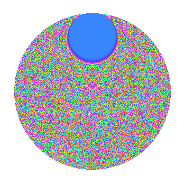# Properties

 Label 2.72.a.bLevel 2 Weight 72 Character orbit 2.a Self dual Yes Analytic conductor 63.849 Analytic rank 0 Dimension 3 CM No Inner twists 1

# Related objects

## Newspace parameters

 Level: $$N$$ = $$2$$ Weight: $$k$$ = $$72$$ Character orbit: $$[\chi]$$ = 2.a (trivial)

## Newform invariants

 Self dual: Yes Analytic conductor: $$63.8492321122$$ Analytic rank: $$0$$ Dimension: $$3$$ Coefficient field: $$\mathbb{Q}[x]/(x^{3} - \cdots)$$ Coefficient ring: $$\Z[a_1, \ldots, a_{5}]$$ Coefficient ring index: $$2^{22}\cdot 3^{6}\cdot 5^{3}\cdot 7$$ Fricke sign: $$1$$ Sato-Tate group: $\mathrm{SU}(2)$

## $q$-expansion

Coefficients of the $$q$$-expansion are expressed in terms of a basis $$1,\beta_1,\beta_2$$ for the coefficient ring described below. We also show the integral $$q$$-expansion of the trace form.

 $$f(q)$$ $$=$$ $$q$$ $$-34359738368 q^{2}$$ $$+(787523430902412 + \beta_{1}) q^{3}$$ $$+$$$$11\!\cdots\!24$$$$q^{4}$$ $$+($$$$15\!\cdots\!50$$$$+ 22397358 \beta_{1} - 3071 \beta_{2}) q^{5}$$ $$+(-$$$$27\!\cdots\!16$$$$- 34359738368 \beta_{1}) q^{6}$$ $$+(-$$$$22\!\cdots\!24$$$$- 8176070633262 \beta_{1} + 180943420 \beta_{2}) q^{7}$$ $$-$$$$40\!\cdots\!32$$$$q^{8}$$ $$+(-$$$$15\!\cdots\!03$$$$- 51420829207969836 \beta_{1} - 428616082170 \beta_{2}) q^{9}$$ $$+O(q^{10})$$ $$q$$ $$-34359738368 q^{2}$$ $$+(787523430902412 + \beta_{1}) q^{3}$$ $$+$$$$11\!\cdots\!24$$$$q^{4}$$ $$+($$$$15\!\cdots\!50$$$$+ 22397358 \beta_{1} - 3071 \beta_{2}) q^{5}$$ $$+(-$$$$27\!\cdots\!16$$$$- 34359738368 \beta_{1}) q^{6}$$ $$+(-$$$$22\!\cdots\!24$$$$- 8176070633262 \beta_{1} + 180943420 \beta_{2}) q^{7}$$ $$-$$$$40\!\cdots\!32$$$$q^{8}$$ $$+(-$$$$15\!\cdots\!03$$$$- 51420829207969836 \beta_{1} - 428616082170 \beta_{2}) q^{9}$$ $$+(-$$$$54\!\cdots\!00$$$$- 769567361014431744 \beta_{1} + 105518756528128 \beta_{2}) q^{10}$$ $$+($$$$13\!\cdots\!52$$$$-$$$$12\!\cdots\!69$$$$\beta_{1} - 2154477364029320 \beta_{2}) q^{11}$$ $$+($$$$92\!\cdots\!88$$$$+$$$$11\!\cdots\!24$$$$\beta_{1}) q^{12}$$ $$+($$$$84\!\cdots\!82$$$$-$$$$10\!\cdots\!58$$$$\beta_{1} - 1606434955399360255 \beta_{2}) q^{13}$$ $$+($$$$78\!\cdots\!32$$$$+$$$$28\!\cdots\!16$$$$\beta_{1} - 6217168570611138560 \beta_{2}) q^{14}$$ $$+($$$$13\!\cdots\!00$$$$+$$$$18\!\cdots\!06$$$$\beta_{1} -$$$$17\!\cdots\!72$$$$\beta_{2}) q^{15}$$ $$+$$$$13\!\cdots\!76$$$$q^{16}$$ $$+($$$$11\!\cdots\!06$$$$-$$$$37\!\cdots\!08$$$$\beta_{1} +$$$$18\!\cdots\!10$$$$\beta_{2}) q^{17}$$ $$+($$$$51\!\cdots\!04$$$$+$$$$17\!\cdots\!48$$$$\beta_{1} +$$$$14\!\cdots\!60$$$$\beta_{2}) q^{18}$$ $$+($$$$94\!\cdots\!40$$$$+$$$$11\!\cdots\!89$$$$\beta_{1} -$$$$35\!\cdots\!20$$$$\beta_{2}) q^{19}$$ $$+($$$$18\!\cdots\!00$$$$+$$$$26\!\cdots\!92$$$$\beta_{1} -$$$$36\!\cdots\!04$$$$\beta_{2}) q^{20}$$ $$+(-$$$$49\!\cdots\!88$$$$+$$$$11\!\cdots\!52$$$$\beta_{1} +$$$$13\!\cdots\!80$$$$\beta_{2}) q^{21}$$ $$+(-$$$$45\!\cdots\!36$$$$+$$$$42\!\cdots\!92$$$$\beta_{1} +$$$$74\!\cdots\!60$$$$\beta_{2}) q^{22}$$ $$+($$$$12\!\cdots\!72$$$$+$$$$29\!\cdots\!66$$$$\beta_{1} -$$$$13\!\cdots\!20$$$$\beta_{2}) q^{23}$$ $$+(-$$$$31\!\cdots\!84$$$$-$$$$40\!\cdots\!32$$$$\beta_{1}) q^{24}$$ $$+($$$$23\!\cdots\!75$$$$+$$$$63\!\cdots\!00$$$$\beta_{1} -$$$$12\!\cdots\!00$$$$\beta_{2}) q^{25}$$ $$+(-$$$$28\!\cdots\!76$$$$+$$$$34\!\cdots\!44$$$$\beta_{1} +$$$$55\!\cdots\!40$$$$\beta_{2}) q^{26}$$ $$+(-$$$$31\!\cdots\!00$$$$-$$$$61\!\cdots\!22$$$$\beta_{1} -$$$$10\!\cdots\!20$$$$\beta_{2}) q^{27}$$ $$+(-$$$$26\!\cdots\!76$$$$-$$$$96\!\cdots\!88$$$$\beta_{1} +$$$$21\!\cdots\!80$$$$\beta_{2}) q^{28}$$ $$+($$$$94\!\cdots\!90$$$$-$$$$29\!\cdots\!82$$$$\beta_{1} -$$$$33\!\cdots\!95$$$$\beta_{2}) q^{29}$$ $$+(-$$$$46\!\cdots\!00$$$$-$$$$62\!\cdots\!08$$$$\beta_{1} +$$$$60\!\cdots\!96$$$$\beta_{2}) q^{30}$$ $$+(-$$$$20\!\cdots\!88$$$$+$$$$52\!\cdots\!52$$$$\beta_{1} +$$$$11\!\cdots\!80$$$$\beta_{2}) q^{31}$$ $$-$$$$47\!\cdots\!68$$$$q^{32}$$ $$+(-$$$$74\!\cdots\!76$$$$+$$$$87\!\cdots\!64$$$$\beta_{1} -$$$$62\!\cdots\!10$$$$\beta_{2}) q^{33}$$ $$+(-$$$$40\!\cdots\!08$$$$+$$$$12\!\cdots\!44$$$$\beta_{1} -$$$$62\!\cdots\!80$$$$\beta_{2}) q^{34}$$ $$+(-$$$$50\!\cdots\!00$$$$-$$$$52\!\cdots\!12$$$$\beta_{1} +$$$$23\!\cdots\!44$$$$\beta_{2}) q^{35}$$ $$+(-$$$$17\!\cdots\!72$$$$-$$$$60\!\cdots\!64$$$$\beta_{1} -$$$$50\!\cdots\!80$$$$\beta_{2}) q^{36}$$ $$+(-$$$$22\!\cdots\!54$$$$-$$$$15\!\cdots\!38$$$$\beta_{1} -$$$$49\!\cdots\!15$$$$\beta_{2}) q^{37}$$ $$+(-$$$$32\!\cdots\!20$$$$-$$$$40\!\cdots\!52$$$$\beta_{1} +$$$$12\!\cdots\!60$$$$\beta_{2}) q^{38}$$ $$+(-$$$$60\!\cdots\!16$$$$+$$$$21\!\cdots\!66$$$$\beta_{1} -$$$$82\!\cdots\!00$$$$\beta_{2}) q^{39}$$ $$+(-$$$$64\!\cdots\!00$$$$-$$$$90\!\cdots\!56$$$$\beta_{1} +$$$$12\!\cdots\!72$$$$\beta_{2}) q^{40}$$ $$+($$$$85\!\cdots\!62$$$$+$$$$12\!\cdots\!08$$$$\beta_{1} +$$$$34\!\cdots\!60$$$$\beta_{2}) q^{41}$$ $$+($$$$16\!\cdots\!84$$$$-$$$$39\!\cdots\!36$$$$\beta_{1} -$$$$45\!\cdots\!40$$$$\beta_{2}) q^{42}$$ $$+($$$$63\!\cdots\!12$$$$+$$$$54\!\cdots\!43$$$$\beta_{1} -$$$$12\!\cdots\!20$$$$\beta_{2}) q^{43}$$ $$+($$$$15\!\cdots\!48$$$$-$$$$14\!\cdots\!56$$$$\beta_{1} -$$$$25\!\cdots\!80$$$$\beta_{2}) q^{44}$$ $$+(-$$$$90\!\cdots\!50$$$$-$$$$47\!\cdots\!34$$$$\beta_{1} +$$$$12\!\cdots\!33$$$$\beta_{2}) q^{45}$$ $$+(-$$$$42\!\cdots\!96$$$$-$$$$10\!\cdots\!88$$$$\beta_{1} +$$$$45\!\cdots\!60$$$$\beta_{2}) q^{46}$$ $$+(-$$$$14\!\cdots\!44$$$$+$$$$31\!\cdots\!68$$$$\beta_{1} -$$$$23\!\cdots\!60$$$$\beta_{2}) q^{47}$$ $$+($$$$10\!\cdots\!12$$$$+$$$$13\!\cdots\!76$$$$\beta_{1}) q^{48}$$ $$+(-$$$$34\!\cdots\!67$$$$+$$$$33\!\cdots\!36$$$$\beta_{1} -$$$$25\!\cdots\!40$$$$\beta_{2}) q^{49}$$ $$+(-$$$$80\!\cdots\!00$$$$-$$$$21\!\cdots\!00$$$$\beta_{1} +$$$$44\!\cdots\!00$$$$\beta_{2}) q^{50}$$ $$+(-$$$$22\!\cdots\!28$$$$+$$$$22\!\cdots\!90$$$$\beta_{1} +$$$$11\!\cdots\!80$$$$\beta_{2}) q^{51}$$ $$+($$$$99\!\cdots\!68$$$$-$$$$11\!\cdots\!92$$$$\beta_{1} -$$$$18\!\cdots\!20$$$$\beta_{2}) q^{52}$$ $$+($$$$10\!\cdots\!02$$$$-$$$$18\!\cdots\!58$$$$\beta_{1} -$$$$26\!\cdots\!35$$$$\beta_{2}) q^{53}$$ $$+($$$$10\!\cdots\!00$$$$+$$$$21\!\cdots\!96$$$$\beta_{1} +$$$$34\!\cdots\!60$$$$\beta_{2}) q^{54}$$ $$+($$$$27\!\cdots\!00$$$$+$$$$20\!\cdots\!26$$$$\beta_{1} +$$$$14\!\cdots\!88$$$$\beta_{2}) q^{55}$$ $$+($$$$92\!\cdots\!68$$$$+$$$$33\!\cdots\!84$$$$\beta_{1} -$$$$73\!\cdots\!40$$$$\beta_{2}) q^{56}$$ $$+($$$$70\!\cdots\!80$$$$+$$$$50\!\cdots\!68$$$$\beta_{1} -$$$$24\!\cdots\!70$$$$\beta_{2}) q^{57}$$ $$+(-$$$$32\!\cdots\!20$$$$+$$$$10\!\cdots\!76$$$$\beta_{1} +$$$$11\!\cdots\!60$$$$\beta_{2}) q^{58}$$ $$+($$$$15\!\cdots\!80$$$$-$$$$10\!\cdots\!89$$$$\beta_{1} -$$$$25\!\cdots\!40$$$$\beta_{2}) q^{59}$$ $$+($$$$16\!\cdots\!00$$$$+$$$$21\!\cdots\!44$$$$\beta_{1} -$$$$20\!\cdots\!28$$$$\beta_{2}) q^{60}$$ $$+($$$$55\!\cdots\!22$$$$+$$$$79\!\cdots\!54$$$$\beta_{1} +$$$$11\!\cdots\!85$$$$\beta_{2}) q^{61}$$ $$+($$$$69\!\cdots\!84$$$$-$$$$17\!\cdots\!36$$$$\beta_{1} -$$$$38\!\cdots\!40$$$$\beta_{2}) q^{62}$$ $$+($$$$23\!\cdots\!72$$$$+$$$$29\!\cdots\!30$$$$\beta_{1} -$$$$69\!\cdots\!20$$$$\beta_{2}) q^{63}$$ $$+$$$$16\!\cdots\!24$$$$q^{64}$$ $$+($$$$31\!\cdots\!00$$$$+$$$$29\!\cdots\!76$$$$\beta_{1} -$$$$30\!\cdots\!12$$$$\beta_{2}) q^{65}$$ $$+($$$$25\!\cdots\!68$$$$-$$$$30\!\cdots\!52$$$$\beta_{1} +$$$$21\!\cdots\!80$$$$\beta_{2}) q^{66}$$ $$+($$$$98\!\cdots\!36$$$$-$$$$21\!\cdots\!31$$$$\beta_{1} +$$$$51\!\cdots\!40$$$$\beta_{2}) q^{67}$$ $$+($$$$13\!\cdots\!44$$$$-$$$$43\!\cdots\!92$$$$\beta_{1} +$$$$21\!\cdots\!40$$$$\beta_{2}) q^{68}$$ $$+($$$$17\!\cdots\!64$$$$-$$$$13\!\cdots\!96$$$$\beta_{1} -$$$$19\!\cdots\!60$$$$\beta_{2}) q^{69}$$ $$+($$$$17\!\cdots\!00$$$$+$$$$18\!\cdots\!16$$$$\beta_{1} -$$$$81\!\cdots\!92$$$$\beta_{2}) q^{70}$$ $$+($$$$30\!\cdots\!32$$$$+$$$$56\!\cdots\!94$$$$\beta_{1} +$$$$28\!\cdots\!80$$$$\beta_{2}) q^{71}$$ $$+($$$$60\!\cdots\!96$$$$+$$$$20\!\cdots\!52$$$$\beta_{1} +$$$$17\!\cdots\!40$$$$\beta_{2}) q^{72}$$ $$+($$$$93\!\cdots\!62$$$$-$$$$13\!\cdots\!28$$$$\beta_{1} +$$$$31\!\cdots\!90$$$$\beta_{2}) q^{73}$$ $$+($$$$78\!\cdots\!72$$$$+$$$$53\!\cdots\!84$$$$\beta_{1} +$$$$16\!\cdots\!20$$$$\beta_{2}) q^{74}$$ $$+($$$$38\!\cdots\!00$$$$-$$$$40\!\cdots\!25$$$$\beta_{1} -$$$$96\!\cdots\!00$$$$\beta_{2}) q^{75}$$ $$+($$$$11\!\cdots\!60$$$$+$$$$13\!\cdots\!36$$$$\beta_{1} -$$$$42\!\cdots\!80$$$$\beta_{2}) q^{76}$$ $$+($$$$32\!\cdots\!52$$$$-$$$$55\!\cdots\!48$$$$\beta_{1} -$$$$13\!\cdots\!40$$$$\beta_{2}) q^{77}$$ $$+($$$$20\!\cdots\!88$$$$-$$$$72\!\cdots\!88$$$$\beta_{1} +$$$$28\!\cdots\!00$$$$\beta_{2}) q^{78}$$ $$+($$$$11\!\cdots\!80$$$$+$$$$18\!\cdots\!44$$$$\beta_{1} +$$$$14\!\cdots\!60$$$$\beta_{2}) q^{79}$$ $$+($$$$22\!\cdots\!00$$$$+$$$$31\!\cdots\!08$$$$\beta_{1} -$$$$42\!\cdots\!96$$$$\beta_{2}) q^{80}$$ $$+(-$$$$25\!\cdots\!59$$$$+$$$$39\!\cdots\!48$$$$\beta_{1} +$$$$57\!\cdots\!90$$$$\beta_{2}) q^{81}$$ $$+(-$$$$29\!\cdots\!16$$$$-$$$$41\!\cdots\!44$$$$\beta_{1} -$$$$11\!\cdots\!80$$$$\beta_{2}) q^{82}$$ $$+(-$$$$16\!\cdots\!88$$$$+$$$$80\!\cdots\!45$$$$\beta_{1} -$$$$16\!\cdots\!60$$$$\beta_{2}) q^{83}$$ $$+(-$$$$58\!\cdots\!12$$$$+$$$$13\!\cdots\!48$$$$\beta_{1} +$$$$15\!\cdots\!20$$$$\beta_{2}) q^{84}$$ $$+(-$$$$38\!\cdots\!00$$$$-$$$$37\!\cdots\!32$$$$\beta_{1} +$$$$52\!\cdots\!34$$$$\beta_{2}) q^{85}$$ $$+(-$$$$21\!\cdots\!16$$$$-$$$$18\!\cdots\!24$$$$\beta_{1} +$$$$43\!\cdots\!60$$$$\beta_{2}) q^{86}$$ $$+(-$$$$17\!\cdots\!20$$$$+$$$$40\!\cdots\!26$$$$\beta_{1} -$$$$16\!\cdots\!00$$$$\beta_{2}) q^{87}$$ $$+(-$$$$53\!\cdots\!64$$$$+$$$$50\!\cdots\!08$$$$\beta_{1} +$$$$87\!\cdots\!40$$$$\beta_{2}) q^{88}$$ $$+(-$$$$59\!\cdots\!90$$$$+$$$$62\!\cdots\!20$$$$\beta_{1} -$$$$72\!\cdots\!50$$$$\beta_{2}) q^{89}$$ $$+($$$$31\!\cdots\!00$$$$+$$$$16\!\cdots\!12$$$$\beta_{1} -$$$$44\!\cdots\!44$$$$\beta_{2}) q^{90}$$ $$+(-$$$$15\!\cdots\!68$$$$-$$$$30\!\cdots\!52$$$$\beta_{1} +$$$$95\!\cdots\!40$$$$\beta_{2}) q^{91}$$ $$+($$$$14\!\cdots\!28$$$$+$$$$34\!\cdots\!84$$$$\beta_{1} -$$$$15\!\cdots\!80$$$$\beta_{2}) q^{92}$$ $$+($$$$29\!\cdots\!44$$$$-$$$$28\!\cdots\!84$$$$\beta_{1} +$$$$57\!\cdots\!20$$$$\beta_{2}) q^{93}$$ $$+($$$$50\!\cdots\!92$$$$-$$$$10\!\cdots\!24$$$$\beta_{1} +$$$$79\!\cdots\!80$$$$\beta_{2}) q^{94}$$ $$+($$$$10\!\cdots\!00$$$$+$$$$10\!\cdots\!10$$$$\beta_{1} -$$$$54\!\cdots\!20$$$$\beta_{2}) q^{95}$$ $$+(-$$$$37\!\cdots\!16$$$$-$$$$47\!\cdots\!68$$$$\beta_{1}) q^{96}$$ $$+($$$$13\!\cdots\!86$$$$+$$$$31\!\cdots\!60$$$$\beta_{1} +$$$$15\!\cdots\!90$$$$\beta_{2}) q^{97}$$ $$+($$$$11\!\cdots\!56$$$$-$$$$11\!\cdots\!48$$$$\beta_{1} +$$$$87\!\cdots\!20$$$$\beta_{2}) q^{98}$$ $$+($$$$42\!\cdots\!44$$$$-$$$$24\!\cdots\!05$$$$\beta_{1} +$$$$90\!\cdots\!40$$$$\beta_{2}) q^{99}$$ $$+O(q^{100})$$ $$\operatorname{Tr}(f)(q)$$ $$=$$ $$3q$$ $$\mathstrut -\mathstrut 103079215104q^{2}$$ $$\mathstrut +\mathstrut 2362570292707236q^{3}$$ $$\mathstrut +\mathstrut$$$$35\!\cdots\!72$$$$q^{4}$$ $$\mathstrut +\mathstrut$$$$47\!\cdots\!50$$$$q^{5}$$ $$\mathstrut -\mathstrut$$$$81\!\cdots\!48$$$$q^{6}$$ $$\mathstrut -\mathstrut$$$$68\!\cdots\!72$$$$q^{7}$$ $$\mathstrut -\mathstrut$$$$12\!\cdots\!96$$$$q^{8}$$ $$\mathstrut -\mathstrut$$$$45\!\cdots\!09$$$$q^{9}$$ $$\mathstrut +\mathstrut O(q^{10})$$ $$3q$$ $$\mathstrut -\mathstrut 103079215104q^{2}$$ $$\mathstrut +\mathstrut 2362570292707236q^{3}$$ $$\mathstrut +\mathstrut$$$$35\!\cdots\!72$$$$q^{4}$$ $$\mathstrut +\mathstrut$$$$47\!\cdots\!50$$$$q^{5}$$ $$\mathstrut -\mathstrut$$$$81\!\cdots\!48$$$$q^{6}$$ $$\mathstrut -\mathstrut$$$$68\!\cdots\!72$$$$q^{7}$$ $$\mathstrut -\mathstrut$$$$12\!\cdots\!96$$$$q^{8}$$ $$\mathstrut -\mathstrut$$$$45\!\cdots\!09$$$$q^{9}$$ $$\mathstrut -\mathstrut$$$$16\!\cdots\!00$$$$q^{10}$$ $$\mathstrut +\mathstrut$$$$39\!\cdots\!56$$$$q^{11}$$ $$\mathstrut +\mathstrut$$$$27\!\cdots\!64$$$$q^{12}$$ $$\mathstrut +\mathstrut$$$$25\!\cdots\!46$$$$q^{13}$$ $$\mathstrut +\mathstrut$$$$23\!\cdots\!96$$$$q^{14}$$ $$\mathstrut +\mathstrut$$$$40\!\cdots\!00$$$$q^{15}$$ $$\mathstrut +\mathstrut$$$$41\!\cdots\!28$$$$q^{16}$$ $$\mathstrut +\mathstrut$$$$35\!\cdots\!18$$$$q^{17}$$ $$\mathstrut +\mathstrut$$$$15\!\cdots\!12$$$$q^{18}$$ $$\mathstrut +\mathstrut$$$$28\!\cdots\!20$$$$q^{19}$$ $$\mathstrut +\mathstrut$$$$56\!\cdots\!00$$$$q^{20}$$ $$\mathstrut -\mathstrut$$$$14\!\cdots\!64$$$$q^{21}$$ $$\mathstrut -\mathstrut$$$$13\!\cdots\!08$$$$q^{22}$$ $$\mathstrut +\mathstrut$$$$37\!\cdots\!16$$$$q^{23}$$ $$\mathstrut -\mathstrut$$$$95\!\cdots\!52$$$$q^{24}$$ $$\mathstrut +\mathstrut$$$$69\!\cdots\!25$$$$q^{25}$$ $$\mathstrut -\mathstrut$$$$86\!\cdots\!28$$$$q^{26}$$ $$\mathstrut -\mathstrut$$$$94\!\cdots\!00$$$$q^{27}$$ $$\mathstrut -\mathstrut$$$$80\!\cdots\!28$$$$q^{28}$$ $$\mathstrut +\mathstrut$$$$28\!\cdots\!70$$$$q^{29}$$ $$\mathstrut -\mathstrut$$$$13\!\cdots\!00$$$$q^{30}$$ $$\mathstrut -\mathstrut$$$$60\!\cdots\!64$$$$q^{31}$$ $$\mathstrut -\mathstrut$$$$14\!\cdots\!04$$$$q^{32}$$ $$\mathstrut -\mathstrut$$$$22\!\cdots\!28$$$$q^{33}$$ $$\mathstrut -\mathstrut$$$$12\!\cdots\!24$$$$q^{34}$$ $$\mathstrut -\mathstrut$$$$15\!\cdots\!00$$$$q^{35}$$ $$\mathstrut -\mathstrut$$$$53\!\cdots\!16$$$$q^{36}$$ $$\mathstrut -\mathstrut$$$$68\!\cdots\!62$$$$q^{37}$$ $$\mathstrut -\mathstrut$$$$97\!\cdots\!60$$$$q^{38}$$ $$\mathstrut -\mathstrut$$$$18\!\cdots\!48$$$$q^{39}$$ $$\mathstrut -\mathstrut$$$$19\!\cdots\!00$$$$q^{40}$$ $$\mathstrut +\mathstrut$$$$25\!\cdots\!86$$$$q^{41}$$ $$\mathstrut +\mathstrut$$$$50\!\cdots\!52$$$$q^{42}$$ $$\mathstrut +\mathstrut$$$$18\!\cdots\!36$$$$q^{43}$$ $$\mathstrut +\mathstrut$$$$46\!\cdots\!44$$$$q^{44}$$ $$\mathstrut -\mathstrut$$$$27\!\cdots\!50$$$$q^{45}$$ $$\mathstrut -\mathstrut$$$$12\!\cdots\!88$$$$q^{46}$$ $$\mathstrut -\mathstrut$$$$43\!\cdots\!32$$$$q^{47}$$ $$\mathstrut +\mathstrut$$$$32\!\cdots\!36$$$$q^{48}$$ $$\mathstrut -\mathstrut$$$$10\!\cdots\!01$$$$q^{49}$$ $$\mathstrut -\mathstrut$$$$24\!\cdots\!00$$$$q^{50}$$ $$\mathstrut -\mathstrut$$$$66\!\cdots\!84$$$$q^{51}$$ $$\mathstrut +\mathstrut$$$$29\!\cdots\!04$$$$q^{52}$$ $$\mathstrut +\mathstrut$$$$30\!\cdots\!06$$$$q^{53}$$ $$\mathstrut +\mathstrut$$$$32\!\cdots\!00$$$$q^{54}$$ $$\mathstrut +\mathstrut$$$$82\!\cdots\!00$$$$q^{55}$$ $$\mathstrut +\mathstrut$$$$27\!\cdots\!04$$$$q^{56}$$ $$\mathstrut +\mathstrut$$$$21\!\cdots\!40$$$$q^{57}$$ $$\mathstrut -\mathstrut$$$$97\!\cdots\!60$$$$q^{58}$$ $$\mathstrut +\mathstrut$$$$46\!\cdots\!40$$$$q^{59}$$ $$\mathstrut +\mathstrut$$$$48\!\cdots\!00$$$$q^{60}$$ $$\mathstrut +\mathstrut$$$$16\!\cdots\!66$$$$q^{61}$$ $$\mathstrut +\mathstrut$$$$20\!\cdots\!52$$$$q^{62}$$ $$\mathstrut +\mathstrut$$$$71\!\cdots\!16$$$$q^{63}$$ $$\mathstrut +\mathstrut$$$$49\!\cdots\!72$$$$q^{64}$$ $$\mathstrut +\mathstrut$$$$94\!\cdots\!00$$$$q^{65}$$ $$\mathstrut +\mathstrut$$$$76\!\cdots\!04$$$$q^{66}$$ $$\mathstrut +\mathstrut$$$$29\!\cdots\!08$$$$q^{67}$$ $$\mathstrut +\mathstrut$$$$41\!\cdots\!32$$$$q^{68}$$ $$\mathstrut +\mathstrut$$$$52\!\cdots\!92$$$$q^{69}$$ $$\mathstrut +\mathstrut$$$$51\!\cdots\!00$$$$q^{70}$$ $$\mathstrut +\mathstrut$$$$91\!\cdots\!96$$$$q^{71}$$ $$\mathstrut +\mathstrut$$$$18\!\cdots\!88$$$$q^{72}$$ $$\mathstrut +\mathstrut$$$$28\!\cdots\!86$$$$q^{73}$$ $$\mathstrut +\mathstrut$$$$23\!\cdots\!16$$$$q^{74}$$ $$\mathstrut +\mathstrut$$$$11\!\cdots\!00$$$$q^{75}$$ $$\mathstrut +\mathstrut$$$$33\!\cdots\!80$$$$q^{76}$$ $$\mathstrut +\mathstrut$$$$98\!\cdots\!56$$$$q^{77}$$ $$\mathstrut +\mathstrut$$$$62\!\cdots\!64$$$$q^{78}$$ $$\mathstrut +\mathstrut$$$$35\!\cdots\!40$$$$q^{79}$$ $$\mathstrut +\mathstrut$$$$66\!\cdots\!00$$$$q^{80}$$ $$\mathstrut -\mathstrut$$$$77\!\cdots\!77$$$$q^{81}$$ $$\mathstrut -\mathstrut$$$$87\!\cdots\!48$$$$q^{82}$$ $$\mathstrut -\mathstrut$$$$49\!\cdots\!64$$$$q^{83}$$ $$\mathstrut -\mathstrut$$$$17\!\cdots\!36$$$$q^{84}$$ $$\mathstrut -\mathstrut$$$$11\!\cdots\!00$$$$q^{85}$$ $$\mathstrut -\mathstrut$$$$64\!\cdots\!48$$$$q^{86}$$ $$\mathstrut -\mathstrut$$$$53\!\cdots\!60$$$$q^{87}$$ $$\mathstrut -\mathstrut$$$$16\!\cdots\!92$$$$q^{88}$$ $$\mathstrut -\mathstrut$$$$17\!\cdots\!70$$$$q^{89}$$ $$\mathstrut +\mathstrut$$$$93\!\cdots\!00$$$$q^{90}$$ $$\mathstrut -\mathstrut$$$$46\!\cdots\!04$$$$q^{91}$$ $$\mathstrut +\mathstrut$$$$44\!\cdots\!84$$$$q^{92}$$ $$\mathstrut +\mathstrut$$$$89\!\cdots\!32$$$$q^{93}$$ $$\mathstrut +\mathstrut$$$$15\!\cdots\!76$$$$q^{94}$$ $$\mathstrut +\mathstrut$$$$30\!\cdots\!00$$$$q^{95}$$ $$\mathstrut -\mathstrut$$$$11\!\cdots\!48$$$$q^{96}$$ $$\mathstrut +\mathstrut$$$$41\!\cdots\!58$$$$q^{97}$$ $$\mathstrut +\mathstrut$$$$35\!\cdots\!68$$$$q^{98}$$ $$\mathstrut +\mathstrut$$$$12\!\cdots\!32$$$$q^{99}$$ $$\mathstrut +\mathstrut O(q^{100})$$

Basis of coefficient ring in terms of a root $$\nu$$ of $$x^{3}\mathstrut -\mathstrut$$ $$71437129084791448795855051$$ $$x\mathstrut -\mathstrut$$ $$180952663419752575975880178936282470070$$:

 $$\beta_{0}$$ $$=$$ $$1$$ $$\beta_{1}$$ $$=$$ $$($$$$-720 \nu^{2} + 4460299047094320 \nu + 34289821960699895422010424480$$$$)/$$$$249228641669$$ $$\beta_{2}$$ $$=$$ $$($$$$18347040 \nu^{2} + 2105974227666339360 \nu - 873773243202554735143669636599360$$$$)/$$$$249228641669$$
 $$1$$ $$=$$ $$\beta_0$$ $$\nu$$ $$=$$ $$($$$$\beta_{2}\mathstrut +\mathstrut$$ $$25482$$ $$\beta_{1}$$$$)/$$$$464486400$$ $$\nu^{2}$$ $$=$$ $$($$$$6194859787631$$ $$\beta_{2}\mathstrut -\mathstrut$$ $$2924964205092138$$ $$\beta_{1}\mathstrut +\mathstrut$$ $$22121049943286716534647365040537600$$$$)/$$$$464486400$$

## Embeddings

For each embedding $$\iota_m$$ of the coefficient field, the values $$\iota_m(a_n)$$ are shown below.

For more information on an embedded modular form you can click on its label.

Label $$\iota_m(\nu)$$ $$a_{2}$$ $$a_{3}$$ $$a_{4}$$ $$a_{5}$$ $$a_{6}$$ $$a_{7}$$ $$a_{8}$$ $$a_{9}$$ $$a_{10}$$
1.1
 −6.65040e12 9.51117e12 −2.86077e12
−3.43597e10 −1.08417e17 1.18059e21 7.90573e22 3.72520e27 6.08855e29 −4.05648e31 4.24488e33 −2.71639e33
1.2 −3.43597e10 4.72491e16 1.18059e21 −7.30618e24 −1.62347e27 −2.33219e28 −4.05648e31 −5.27699e33 2.51038e35
1.3 −3.43597e10 6.35309e16 1.18059e21 1.19804e25 −2.18290e27 −1.27132e30 −4.05648e31 −3.47329e33 −4.11644e35
 $$n$$: e.g. 2-40 or 990-1000 Significant digits: Format: Complex embeddings Normalized embeddings Satake parameters Satake angles

## Inner twists

This newform does not admit any (nontrivial) inner twists.

## Atkin-Lehner signs

$$p$$ Sign
$$2$$ $$1$$

## Hecke kernels

This newform can be constructed as the kernel of the linear operator $$T_{3}^{3}$$ $$\mathstrut -\mathstrut$$$$23\!\cdots\!36$$$$T_{3}^{2}$$ $$\mathstrut -\mathstrut$$$$90\!\cdots\!68$$$$T_{3}$$ $$\mathstrut +\mathstrut$$$$32\!\cdots\!72$$ acting on $$S_{72}^{\mathrm{new}}(\Gamma_0(2))$$.